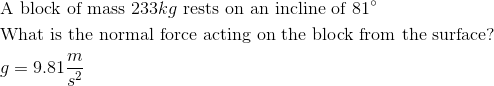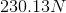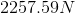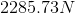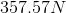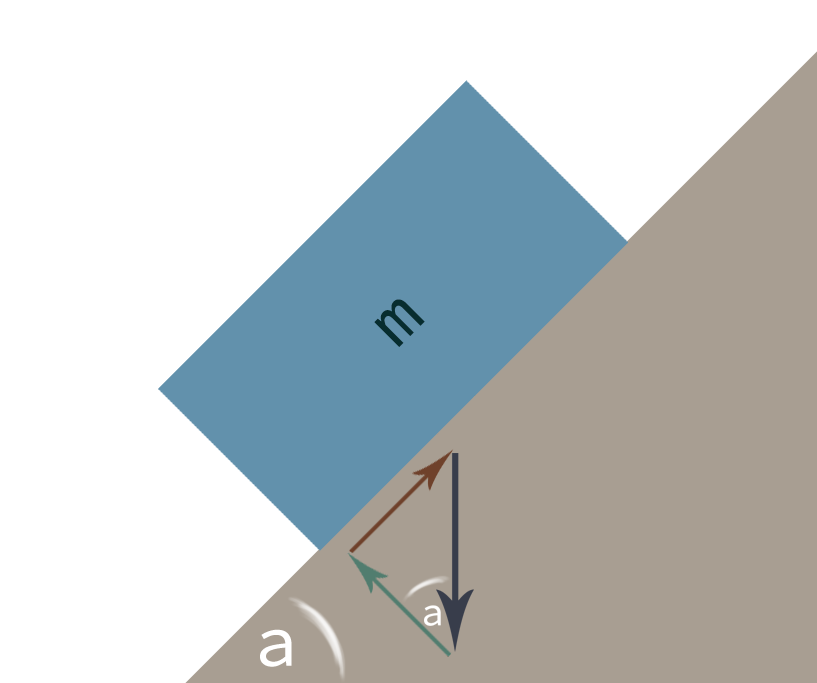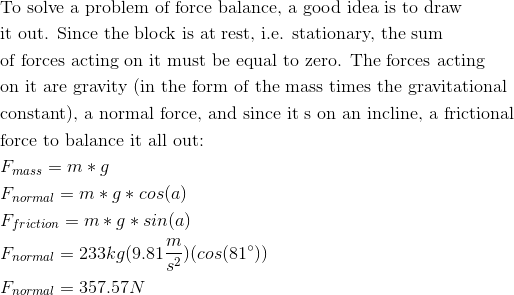AP Physics C Electricity : Mechanics Exam

Example Questions

Example Question #71 : Motion

An ice skater begins to spin, starting with his arms spread out as far as possible, parallel to the ice. He pulls his arms into his body, and then raises them completely vertically towards the ceiling. As the skater pulls his arms inward, his angular velocity will __________, and as he raises his arms vertically his angular velocity will __________.

increase . . . decrease

decrease . . . increase

increase . . . stay the same

decrease . . . stay the same

increase . . . stay the same

Explanation:

Through conservation of angular momentum, as moment of inertia decreases, the angular velocity increases. Moment of inertia is dependent on the distribution of the spinning body's mass away from the center of mass—as the skater brings his arms in, he lowers his moment of inertia, thus increasing his angular velocity. If he raises his arms completely vertically from this point, it does not change the radius of mass distributions (from the center of his body), thus maintaining his angular velocity.

Example Question #71 : Motion

Sarah spins a ball of massattached to a string of lengtharound her head with a velocity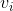. If the ball splits in half, losing exactly one-half of its mass instantaneously, what is its new velocity,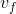?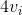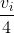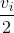(no change in velocity)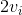Explanation:

By the conservation of angular momentum, the angular momentum, is equal to the product of the mass, angular velocity, and radius (or length of the rope in this case). The equation relating these terms is: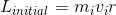Here,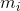is the initial mass,is the initial angular velocity, andis the length of the rope, which remains constant. Angular momentum must be conserved, thus: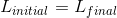Substitute.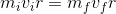We are given that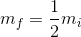andis the final velocity. Plug in and solve.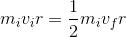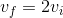Example Question #73 : Motion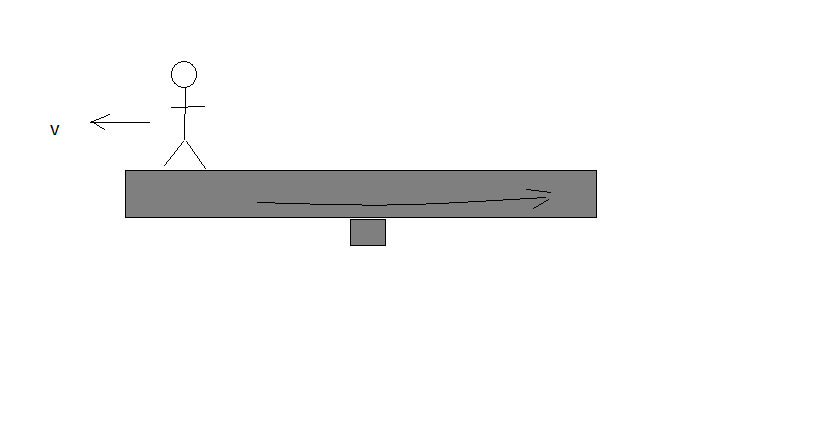A child is standing at the center of a frictionless, rotating platform. Both the child and the platform are rotating with and initial angular velocity,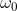. The child begins to walk slowly toward the edge of the platform. Which quantity will decrease as the child walks?

Total angular inertia

Total angular momentum

Total momentum

Rotational inertia of the disk

Total rotational kinetic energy

Total rotational kinetic energy

Explanation:

Since the platform is frictionless, as the child walks, angular momentum is conserved. However, since there is an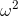term in the kinetic energy expression, as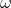decreased due to the increase of inertia, it affects the energy more, and it decreases. From the reference frame of the disk, the child feels an outward directed force, and thus is doing negative work. This is the same principle that allows ice skaters to increase their spinning speed.

Example Question #71 : Ap Physics C

Two solid cylinderical disks have equal radii. The first disk is spinning clockwise at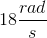and the second disk is spinning counterclockwise at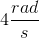. The second disk has a mass three times larger than the first. If both spinning disks are combined to form one disk, they end up rotating at the same angular velocity and same direction. Find this angular velocity after combination.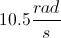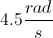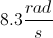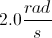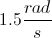Explanation:

For the first disk, we have the information below.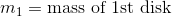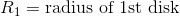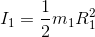For the second disk, we have the information below.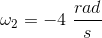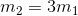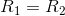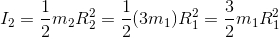To do this problem, we use conservation of angular momentum. Before the disks are put in contact, the initial total angular momentum is given by the equation below.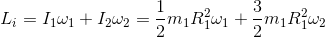It is just the sum of the angular momentums of each disk. When the disks are combined together, then final angular momentum can be found by the following equation.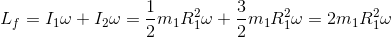Set this equal to the initial angular momentum.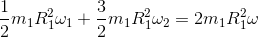Simplify and solve for.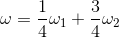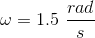Example Question #71 : Motion

A piece of space debris is travelling in an elliptical orbit around a planet. At its closest point to the planet, the debris is travelling at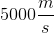. When the debris is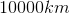from the planet, it travels at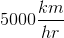. How close does the debris get to the planet in its orbit?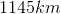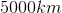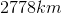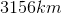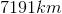Explanation:

The equation for conservation of angular momentum is: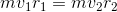This means that the angular momentum of the object at the two points in its orbit must be the same. Since the mass of the debris does not change, this gives us an equality of the product of the velocity and distance of the debris at any two points of its orbit: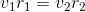Plug in known values.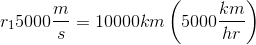There is disagreement between units; the velocities are given in both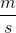and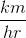. Glance down at the answer choices and note that they are all lengths in kilometers.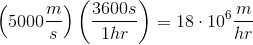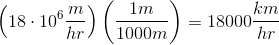Solve for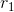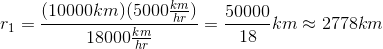Example Question #71 : Ap Physics C

A mechanic is using a wrench to loosen and tighten screws on an engine block and wants to increase the amount of torque he puts on the screws to adjust them more easily. Which of the following steps will not help him to do so?

Pulling or pushing at an angle that is less perpendicular to the wrench

Increasing the magnitude of the force he puts on the wrench

Pulling or pushing at an angle that is more perpendicular to the wrench

Using a longer wrench

Using a wrench of a different material

Pulling or pushing at an angle that is less perpendicular to the wrench

Explanation:

Remember the torque equation: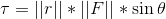Exerting force on the wrench at an angle less perpendicular to the wrench will reduce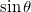and thus reduce the torque.

The other answer options will all increase the torque being applied, making the twisting motion easier.

Example Question #1 : Friction And Normal Force

Weightlessness is experienced when the normal force equals __________.

zero

a negative value

the force due to gravity

the mass of the object

zero

Explanation:

"Weightlessness" is analogous to free-fall (neglecting air resistance), during which the only force on an object is the force of gravity. When normal force becomes zero, the object loses physical contact with the surface.

When normal force is equal to the force of gravity, the object is in equilibrium and the net vertical force is zero. This results in zero acceleration, but does not result in "weightlessness." Mass is not a force, and negative force values merely indicate relative direction (assuming correct calculations)

Example Question #2 : Friction And Normal Force

A book of mass 1kg is held to a vertical wall by a person's hand applying a 20N force directly toward the wall. The wall has a static friction coefficient of 0.3 and a kinetic friction coefficient of 0.2. With the book held at rest, what the is frictional force keeping the book from sliding down the wall?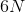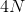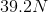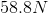Explanation:

Frictional force is calculated using the normal force and the friction coefficient. Since the book is at rest (not in motion), we use the coefficient of static friction.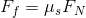The normal force will be equal and opposite the force pushing directly into the surface; in this case, that means the normal force will be 20N.

Solve for the force of friction: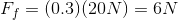Example Question #1 : Normal Force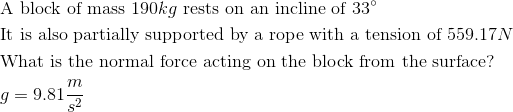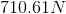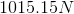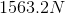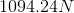Explanation: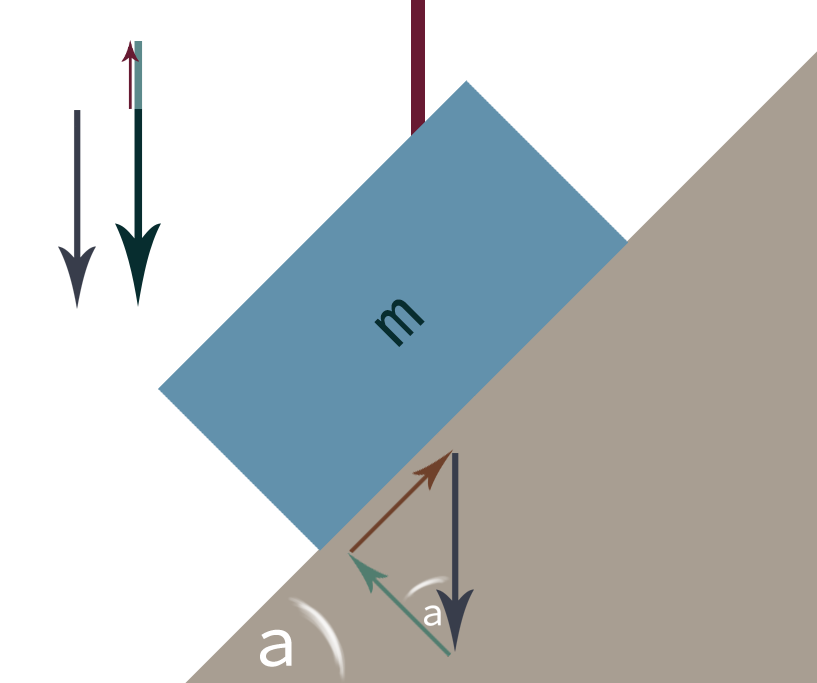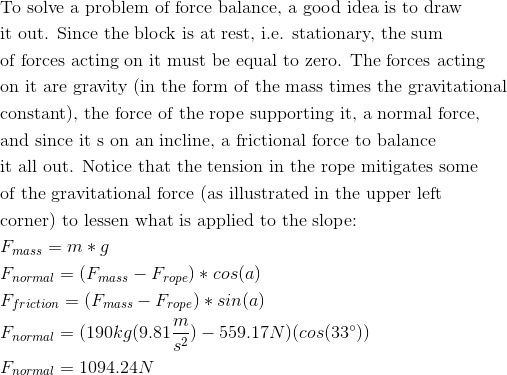Example Question #1 : Forces